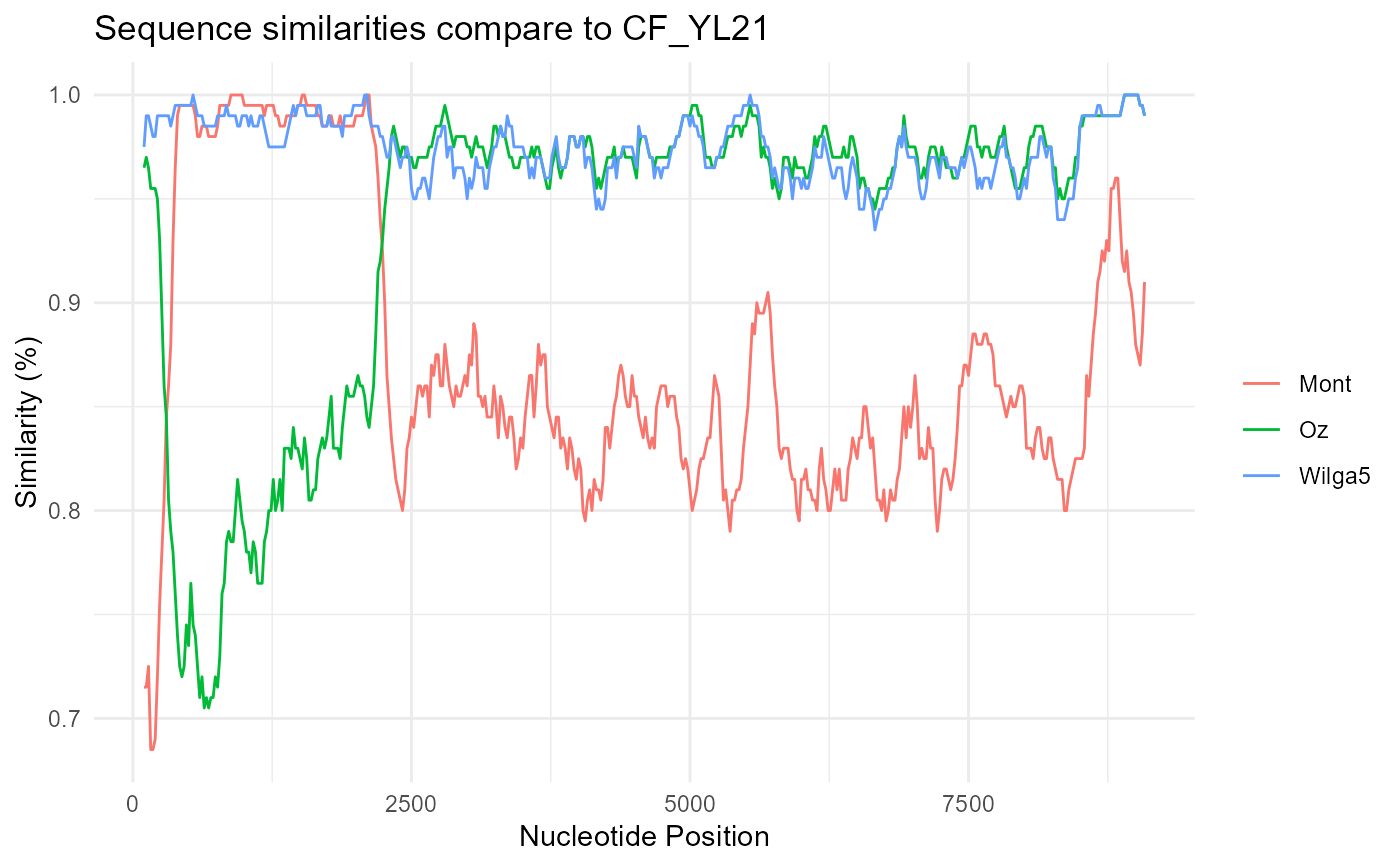Sequence similarity plot

simplot(
file,
query,
window = 200,
step = 20,
group = FALSE,
id,
sep,
sd = FALSE
)

## Arguments

file alignment fast file query sequence sliding window size (bp) step size to slide the window (bp) whether grouping sequence position to extract id for grouping; only works if group = TRUE separator to split sequence name; only works if group = TRUE whether display standard deviation of similarity among each group; only works if group=TRUE

ggplot object

guangchuang yu

## Examples

fas <- system.file("extdata/GVariation/sample_alignment.fa", package="ggmsa")
simplot(fas, 'CF_YL21')